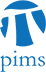## Perfect matchings in grid graphs after vertex deletions

• Date: 12/01/2009
Lecturer(s):
Jonathan Blackman (UBC)
Location:

University of British Columbia

Description:

We investigate the d-dimensional grid graph [m]x[m]x...x[m]

for even m. The graph is bipartite. If we choose a subset B' of the

black vertices and a subset W' of the white vertices then the graph

obtained by deleting B' and W' has a perfect matching if it satisfies

the following conditions

1)|B'|=|W'|

2) each pair x,y in B' are at distance at least c.m^{1/d}

3) each pair x,y in W' are at distance at least c.m^{1/d}

A value for c is given as a function of d. The factor m^{1/d} is in

some sense best possible.

Schedule:

4:00pm - 5:00pm, WMAX 216.